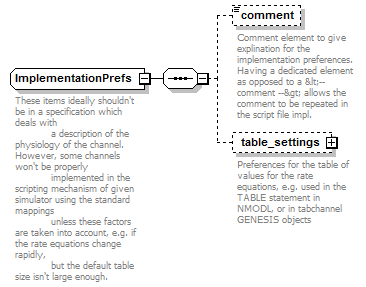# ImplementationPrefs

 ```These items ideally shouldn't be in a specification which deals with a description of the physiology of the channel. However, some channels won't be properly implemented in the scripting mechanism of given simulator using the standard mappings unless these factors are taken into account, e.g. if the rate equations change rapidly, but the default table size isn't large enough.```

## Model## Used By

 Element ChannelType/impl_prefs

## Source

 ``` These items ideally shouldn't be in a specification which deals with a description of the physiology of the channel. However, some channels won't be properly implemented in the scripting mechanism of given simulator using the standard mappings unless these factors are taken into account, e.g. if the rate equations change rapidly, but the default table size isn't large enough. Comment element to give explination for the implementation preferences. Having a dedicated element as opposed to a <-- comment --> allows the comment to be repeated in the script file impl. Preferences for the table of values for the rate equations, e.g. used in the TABLE statement in NMODL, or in tabchannel GENESIS objects The maximum potential from which to calculate the tables of rate values The minimum potential from which to calculate the tables of rate values The number of divisions in the table ```